PHY294H - Lecture 15

For the next three lectures we shall discuss direct current circuits. Our work on electrostatics enables us to understand what a capacitor is and our work on the resistivity of materials enables us to understand the processes which are occuring in resistors. We also need to have a source of applied current and voltage. We could use capacitors for this purpose, but they are rather poor for storing energy, so they aren't usually used, except in cases where a very large, but very short current pulse is required. Our voltage sources are usually chemical in origin, as are most of the stored energy devices in technology. They are called a battery, a DC voltage source or an electromotive force (emf). In the ideal case, which we shall almost always use, the emf is assumed to have a fixed potential, and that it is able to deliver an unlimited amount of current. The symbol we shall use for source emf is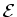. In our circuits the emfwill be fixed, however the current that it supplies depends on the load in the circuit. The load in the circuit is the set of resistors and capacitors to which the emf is supplying current. Our task is to figure out the transient and steady state current supplied to the load for a variety of possible circuits.

An emf and a resistor

An ideal emf supplies a voltage. Let us assume that the positive terminal of the emf is at voltage V1 and is attached by a zero resistance wire to one terminal of a resistor. The negative terminal of the emf is connected, by a zero resistance wire, to the other terminal of the resistor. Since the connecting wires have zero resistance, there is no voltage drop across them. Therefor the voltage at the positive end of the resistor is the same as that of the positive terminal of the emf. Similarly the potential at the negative end of the resistor is the same as that of the negative terminal of the emf. The voltage drop across the resistor is thus. From Ohm's law we have V=IR, so we have,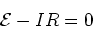(1)

In real life, emf's have an internal resistance, r so no matter how large the load resistance R, there is some dissipation in the emf itself (that is why batteries get hot when you use them). The internal resistance is in series with the load resistance.

Series and parallel combinations of resistors

Series combination

Consider a series combination of resistors R1, R2, ..., Rn. We apply an emf. We want to replace this series combination by one resistor Rseries. Since the same current must flow through all of the resistors (current conservation) and using Ohm's law, we have,(2)

Thus,

 Rseries = R1 + R2 + R3 + .... + Rn (3)

Example

The case above of an emfwith resistance r and load resistance Rleads to an effective emf R+r which is connected in series with the ideal emf. The current flowing in the circuit is then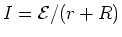. The voltage drop across the internal resistance is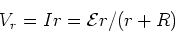(4)

Parallel combination

Consider a parallel combination of resistors R1, R2, ...., Rn. We want to replace this parallel combination by one resistor Rparallel. To do this, note that the voltage across each of these resistors must be the same and it must be equal to. The current supplied to the resistance satisfies. This current must be equal to the sum of the currents passing through the n parallel resistors,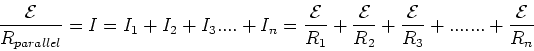(5)

From this we find the parallel resistor combination rule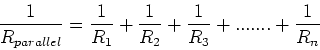(6)

General multiloop circuits

The series and parallel combination laws for resistance and for capacitance are very useful. However they are not the whole story as there are circuits which cannot be reduced using those rules alone. Now we will learn how to solve for the current flow everywhere in multiloop circuits and the voltage drop across all of the resistors and capacitors in our circuits. To carry out this analysis we shall use Kirchhoff's laws:

Kirchhoff's junction rule

The sum of the currents which enter a junction must equal the sum of the currents which leave that junction. (This is the same as current conservation)

Kirchhoff's loop rule

The sum of the voltage drops around a closed loop must be zero. (This is the same are requiing that if we go around a closed loop the amount we go up in potential must be equal to the amount we go down in potential)